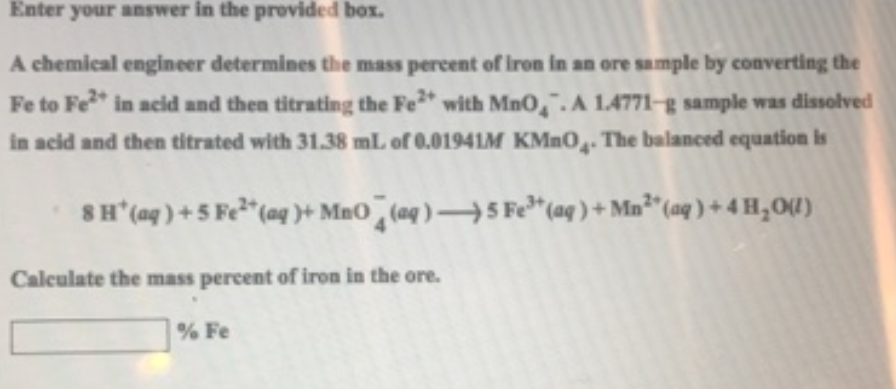# Problem: Enter your answer in the provided box.A chemical engineer determines the mass percent of iron in an ore sample by converting the Fe to Fe2+ in acid and then titrating the Fe 2+ with MnO4-.  A 1.4771-g sample was dissolved in acid and then titrated with 31.38 mL of 0.0194M KMnO4. The balanced equation is 8H+(aq) + 5Fe2+(aq) + MnO4-(aq) → 5Fe3+(aq) + Mn2+(aq) + 4H2O(l) Calculate the mass percent of iron in the ore.

###### FREE Expert Solution
88% (471 ratings)###### Problem Details

A chemical engineer determines the mass percent of iron in an ore sample by converting the Fe to Fe2+ in acid and then titrating the Fe 2+ with MnO4-.  A 1.4771-g sample was dissolved in acid and then titrated with 31.38 mL of 0.0194M KMnO4. The balanced equation is

8H+(aq) + 5Fe2+(aq) + MnO4-(aq) → 5Fe3+(aq) + Mn2+(aq) + 4H2O(l)

Calculate the mass percent of iron in the ore.